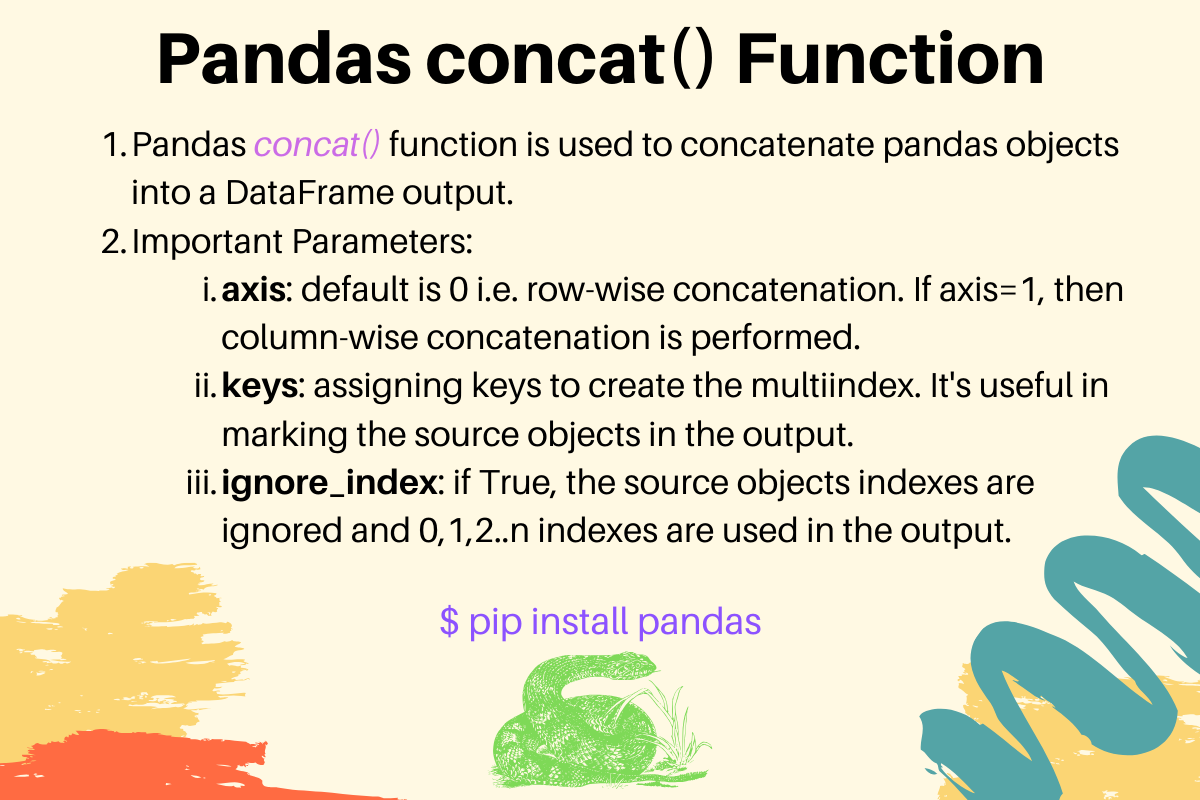# Pandas concat() Examples

Published on August 3, 2022By Pankaj
Developer and author at DigitalOcean.While we believe that this content benefits our community, we have not yet thoroughly reviewed it. If you have any suggestions for improvements, please let us know by clicking the “report an issue“ button at the bottom of the tutorial.

Pandas concat() method is used to concatenate pandas objects such as DataFrames and Series. We can pass various parameters to change the behavior of the concatenation operation.

## 1. Pandas concat() Syntax

The concat() method syntax is:

``````concat(objs, axis=0, join='outer', join_axes=None, ignore_index=False,
keys=None, levels=None, names=None, verify_integrity=False,
sort=None, copy=True)
``````
• objs: a sequence of pandas objects to concatenate.
• join: optional parameter to define how to handle the indexes on the other axis. The valid values are ‘inner’ and ‘outer’.
• join_axes: deprecated in version 0.25.0.
• ignore_index: if True, the indexes from the source objects will be ignored and a sequence of indexes from 0,1,2…n will be assigned to the result.
• keys: a sequence to add an identifier to the result indexes. It’s helpful in marking the source objects in the output.
• levels: a sequence to specify the unique levels to create multiindex.
• names: names for the levels in the resulting hierarchical index.
• verify_integrity: Check whether the new concatenated axis contains duplicates. It’s an expensive operation.
• sort: Sort non-concatenation axis if it is not already aligned when join is ‘outer’. Added in version 0.23.0
• copy: if False, don’t copy data unnecessarily.

## 2. Pandas concat() Example

Let’s look at a simple example to concatenate two DataFrame objects.

``````import pandas

d1 = {"Name": ["Pankaj", "Lisa"], "ID": [1, 2]}
d2 = {"Name": "David", "ID": 3}

df1 = pandas.DataFrame(d1, index={1, 2})
df2 = pandas.DataFrame(d2, index={3})

print('********\n', df1)
print('********\n', df2)

df3 = pandas.concat([df1, df2])

print('********\n', df3)
``````

Output:

``````********
Name  ID
1  Pankaj   1
2    Lisa   2
********
Name  ID
3  David   3
********
Name  ID
1  Pankaj   1
2    Lisa   2
3   David   3
``````

Notice that the concatenation is performed row-wise i.e. 0-axis. Also, the indexes from the source DataFrame objects are preserved in the output.

## 3. Concatenating Along Column i.e. 1-axis

``````d1 = {"Name": ["Pankaj", "Lisa"], "ID": [1, 2]}

df1 = pandas.DataFrame(d1, index={1, 2})
df2 = pandas.DataFrame(d2, index={1, 2})

df3 = pandas.concat([df1, df2], axis=1)
print('********\n', df3)
``````

Output:

``````********
Name  ID    Role
2    Lisa   2  Editor
``````

The concatenation along column makes sense when the source objects contain different kinds of data of an object.

## 4. Assigning Keys to the Concatenated DataFrame Indexes

``````d1 = {"Name": ["Pankaj", "Lisa"], "ID": [1, 2]}
d2 = {"Name": "David", "ID": 3}

df1 = pandas.DataFrame(d1, index={1, 2})
df2 = pandas.DataFrame(d2, index={3})

df3 = pandas.concat([df1, df2], keys=["DF1", "DF2"])
print('********\n', df3)
``````

Output:

``````********
Name  ID
DF1 1  Pankaj   1
2    Lisa   2
DF2 3   David   3
``````

## 5. Ignore Source DataFrame Objects in Concatenation

``````d1 = {"Name": ["Pankaj", "Lisa"], "ID": [1, 2]}
d2 = {"Name": "David", "ID": 3}

df1 = pandas.DataFrame(d1, index={10, 20})
df2 = pandas.DataFrame(d2, index={30})

df3 = pandas.concat([df1, df2], ignore_index=True)
print('********\n', df3)
``````

Output:

``````********
Name  ID
0  Pankaj   1
1    Lisa   2
2   David   3
``````

This is useful when the indexes in the source objects don’t make much sense. So we can ignore them and assign the default indexes to the output DataFrame.

## 6. References

Thanks for learning with the DigitalOcean Community. Check out our offerings for compute, storage, networking, and managed databases.Pankaj

author

Developer and author at DigitalOcean.

#### Still looking for an answer?

Ask a questionSearch for more help

JournalDev
DigitalOcean EmployeeFebruary 2, 2021

Hi Pankaj, thanks for the work. One bit I don’t get. In 4. Assigning Keys to the Concatenated DataFrame Indexes, the index on the far left column shows DF1, then blank, then DF2. ******** Name ID DF1 1 Pankaj 1 2 Lisa 2 DF2 3 David 3 I don’t get the value of leaving the second row blank. How would i search on that? If i ran a query to gather all rows from the d1 data using the far left index col as a the column to search in, it would miss the second row. I don’t understand why anyone would do this. Thanks.

- Stu David

JournalDev
DigitalOcean EmployeeJuly 27, 2020

Hi ! i want to use concatenate function for each row of 2 or most column of my dataset in pandas. Ex: i have a series with 3 columns (NAme, Age , country ) of 10 rows (person). So I want to create a new column which concatenate for each person his name, age and country like (David22USA) Thank for your help.

- Soufiane CAMARA